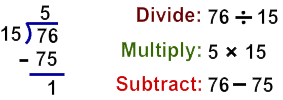# Divide by Two-Digit Numbers (within 100)

Rate 0 stars Common Core
Quiz size:
Message preview:
Someone you know has shared Divide by Two-Digit Numbers (within 100) quiz with you:

To play this quiz, click on the link below:

https://www.turtlediary.com/quiz/divide-by-two-digit-numbers-within-100.html

Hope you have a good experience with this site and recommend to your friends too.

Login to rate activities and track progress.
Login to rate activities and track progress.

Let's find the quotient and remainder when 76 by 15.

In order to divide the given numbers, let’s look at the first two digits.

76 ÷ 15 =  ?

The first two digits are greater than 15.So, 76 ÷ 15 = 5 R 1.

ds

A B C D E F G H I J K L M N O P Q R S T U V W X Y Z

### Help

##### Remember :

The smallest number is the one that comes first while counting.

##### Solution :

To arrange the given numbers in order from smallest to greatest, find the smallest number among all the given numbers.

21,27,23

21 is the smallest number.